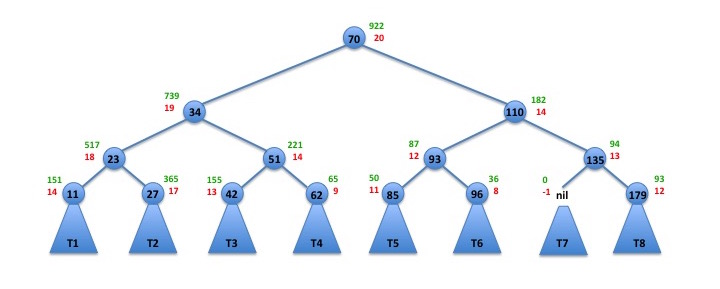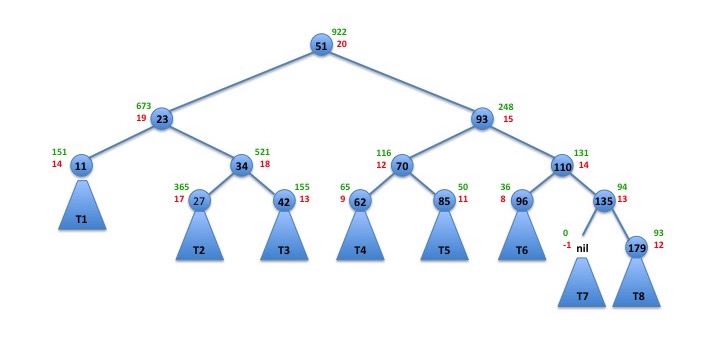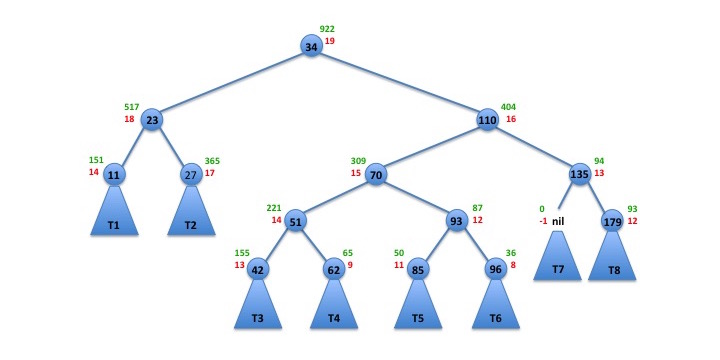# Memory partitioning assignment help provided at a low price

Are you stuck on your memory partitioning assignments? Maybe it’s time you sought professional help. Our programming experts provide help with memory partitioning assignments to college and university students at an affordable price.

## Memory Partioning

If you need help with C++ project, or you need personal online tutoring in C++ then we can help.

If you are looking for a C++ tutor or want someone to help with C++ project then we can provide a solution.

#### Memory Partioning

Simulate the following jobs given the following memory block configuration:• You are going to use Fixed Partition memory scheme.
• Follow the Best-Fit allocation method.
• Calculate the event-driven simulation until all jobs have been executed with the memory as is (in order by address).
• Job sizes are in order of bytes.
Final Output should reflect the following columns.• Note that he above a simply snapshot of your simulation and not the complete table where all jobs have completed.
• Individual submission
Solution: If you want help with your C++ assignment then check out this example.

## Partition.c

• ```#include
#include
#define false 0
#define true 1

int jobTime[] = {5,4,8,2,2,6,8,10,7,6,5,8,9,10,10,7,3,1,9,3,7,2,9,5,10};
int jobSize[] = {5760, 4190, 3290, 2030, 2550, 6990, 8940, 740, 3930, 6890, 6580, 3820, 9140,
420, 220, 7540, 3210, 1380, 9850, 3610, 7540, 2710, 8390, 5950, 760};
int memorySize[] = {9500, 7000, 4500, 8500, 3000, 9000, 1000, 5500, 1500, 500};
int timeLeft = {0}; // store the job's time left
int memIndex = {0}; //store the index of the job that's in memory block
char* status={""};
int jobComplete = {0}, space = 10;
int waitTime={0}, compTime={0}; // wait time and completion time

//update the waiting time of all the jobs
void setWaitTime() {
int  i;
for(i=0;i<25;++i) {
if(status[i] == "free" || status[i] == "waiting") {
waitTime[i]++;
}
}
}

//calculate the best fit for the job, given the job's id
int bestfit(int no) {
int i;
int index = -1;
int job = jobSize[no];
int fits = 0;
for(i=0;i<10;++i) {
if(memorySize[i] >= job) {
if(timeLeft[i] == 0) {  // check if the memory block is free
if(index == -1) {   // check if there's a previous memory slot that can run the current job
index = i;
}
else {
if(memorySize[i] < memorySize[index]) {
index = i;
}
}

}
fits++;
}
}
if(index == -1) {
status[no] = "waiting";
if(fits == 0) {
status[no] = "incomplete";
jobComplete[no] = 1;
}
return -1;
}
timeLeft[index] = jobTime[no];
memIndex[index] = no;
status[no] = "running";
return 1;
}

//allocate jobs to the set of memories that are free and can process the job
void allocateMemory() {
int i;
//
for(i=0;i<25 && space > 0;++i) {
if(jobComplete[i] == false) {
int stat = bestfit(i);
if(stat == 1) {
space --;
}
}
}
//check if the job is completed and update it's status
for(i=0;i<10;++i) {
if(timeLeft[i] > 0) {
timeLeft[i] --;
if(timeLeft[i] == 0) {  // the job is done executing
int no = memIndex[i];
jobComplete[no] = true;
compTime[no] = waitTime[no] + jobTime[no];
status[no] = "done";
space++;
}
}
}
}

//start adding jobs to the memory and wait for all the jobs to get processed
void processJobs() {
int time = 0;
while(true) {
allocateMemory();
setWaitTime();
if(space == 10) {    // all the memory slots are free and all the jobs are completed
break;
}
time++;
}
}

//set status of all the jobs before going into the memory queue
void setStatus() {
int i;
for(i=0;i<25;++i) {
status[i] = "free";
}
}

//print the final result that includes the job's size, runtime and completion time
void print() {
printf("Job\t\t\t\t\t\t\tWait\tCompletion\n");
printf("number\tRun time\tJob size\tJob status\ttime\ttime\n");
int i;
for(i=0;i<25;++i) {
printf("%02d\t%02d\t\t%03d\t\t%10s\t%02d\t%02d\n", i+1, jobTime[i], jobSize[i]/10, status[i], waitTime[i], compTime[i] );
}
printf("\n");
}

int main() {
setStatus();
processJobs();
print();
return 0;
}```
``

#### Index:

• Memory Partioning
• Partition.c
`You may contact us to provide the C++ project help that you need.`